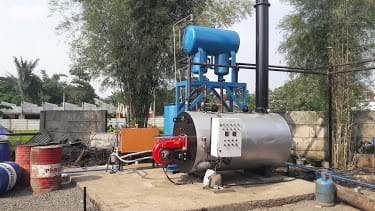## Calculate Boiler Efficiency

• Next Post
• Previous Post# Calculate IDM Boiler Efficiency

The Calculate IDM Boiler efficiency of a boiler is a quantity that shows the relationship between the supply of energy into the boiler and the output energy produced by the boiler. However, the efficiency of the boiler can be defined in three ways, namely:

1. Combustion Efficiency
2. Thermal Efficiency
3. Fuel Efficiency-Steam ( Fuel-to-Steam )

Boiler combustion efficiency generally explains the ability of a burner to burn the entire fuel that goes into the boiler furnace . This type of efficiency is calculated from the amount of fuel that is not burned together with the amount of residual combustion air ( excess water ). The boiler combustion can be said to be efficient if there is no fuel left at the end of the boiler combustion chamber, as well as the amount of residual air.

To get a high combustion efficiency, the burner and boiler combustion chamber must be designed as optimal as possible. On the other hand differences in the use of fuel types also affect combustion efficiency. It is known that liquid fuels and gases (such as LNG and HSD) produce higher combustion efficiency when compared to solid fuels such as coal.

### Calculate Boiler Efficiency

Calculating the combustion efficiency of a boiler is not difficult, we only need to reduce the total amount of heat energy released by combustion with heat energy that passes through the stack (chimney), divided by the total heat energy.

The only thing that is difficult from combustion efficiency is how to pursue the most optimal number. Combustion efficiency is characterized by the burning of the entire fuel in the combustion chamber. While the control parameters used to ensure the overall fuel is burning, is the amount of excess air ( excess air ) that comes out through the stack . The more the amount of excess water that passes through the chimney, the smaller the possibility of the amount of unburned fuel passing through the chimney.

But also, the more the amount of excess waterwhich passes through the chimney, the amount of heat energy escaped by the remaining air is also increasing. Therefore, there is an optimum number of excess air , so that the boiler boiler combustion efficiency is the most optimal.

It appears in the graphic illustration above that the higher the amount of air (oxygen) that passes through the stack , the smaller the amount of fuel including carbon monoxide that has not been completely burned. But also as we discussed above, the higher the amount of excess water , the graph of combustion efficiency falls back down, not least because of the heat energy that escapes with the remaining air.

Then it can be ascertained there is the most optimum value of excess water so that the best combustion efficiency is obtained. As an illustration, the optimum value of excess water for combustion of natural gas is 5 to 10%, liquid fuels are in the number 5 to 20%, and 15 to 60% for coal combustion.

### Calculate Boiler Efficiency

Thermal Efficiency Boiler shows how the boiler performance in terms of its function as a heat exchanger . This efficiency calculation will show how effectively the transfer of heat energy from the combustion process of fuel to water. However, the efficiency calculation is not very accurate, because it does not take into account the loss of radiation heat or convection that is not absorbed by water. In addition, the calculation of boiler thermal efficiency cannot be used for economic analysis, because this calculation does not pay close attention to the amount of fuel consumed. It is on this basis that we will not discuss more deeply about the calculation of boiler thermal efficiency.